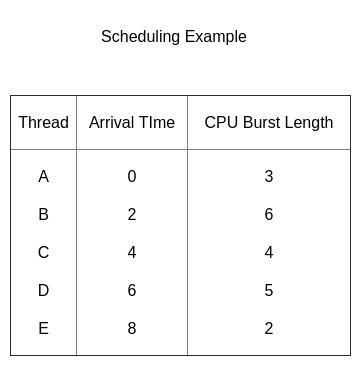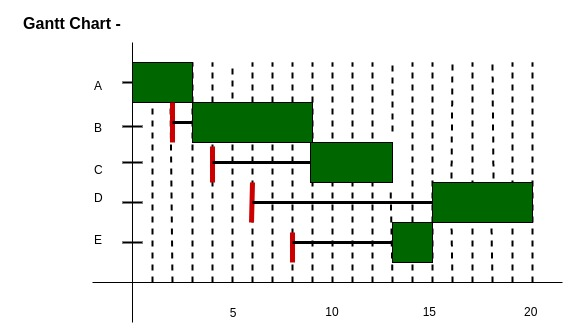Related Articles

# Highest Response Ratio Next (HRRN) CPU Scheduling

• Difficulty Level : Easy
• Last Updated : 14 Jul, 2021

Prerequisite – CPU Scheduling
Given n processes with their Arrival times and Burst times, the task is to find average waiting time and average turn around time using HRRN scheduling algorithm.
The name itself states that we need to find the response ratio of all available processes and select the one with the highest Response Ratio. A process once selected will run till completion.

Criteria – Response Ratio
Mode – Non-Preemptive

` Response Ratio = (W + S)/S`

Here, W is the waiting time of the process so far and S is the Burst time of the process.

Performance of HRRN –

1. Shorter Processes are favoured.
2. Aging without service increases ratio, longer jobs can get past shorter jobs.Gantt Chart –Explanation –

• At t = 0 we have only one process available, so A gets scheduled.
• Similarly, at t = 3 we have only one process available, so B gets scheduled.
• Now at t = 9 we have 3 processes available, C, D and E. Since, C, D and E were available after 4, 6 and 8 units respectively. Therefore, waiting time for C, D and E are (9 – 4 =)5, (9 – 6 =)3, and (9 – 8 =)1 unit respectively.
• Using the formula given above we calculate the Response Ratios of C, D and E respectively as 2.25, 1.6 and 1.5.
• Clearly, C has the highest Response Ratio and so it gets scheduled
• Next at t = 13 we have 2 jobs available D and E.
• Response Ratios of D and E are 2.4 and 3.5 respectively.
• So process E is selected next and process D is selected last.

Implementation of HRRN Scheduling –

1. Input the number of processes, their arrival times and burst times.
2. Sort them according to their arrival times.
3. At any given time calculate the response ratios and select the appropriate process to be scheduled.
4. Calculate the turn around time as completion time – arrival time.
5. Calculate the waiting time as turn around time – burst time.
6. Turn around time divided by the burst time gives the normalized turn around time.
7. Sum up the waiting and turn around times of all processes and divide by the number of processes to get the average waiting and turn around time.

Below is the implementation of above approach:

## C++

 `// C++ program for Highest Response Ratio Next (HRRN) Scheduling``#include ``using` `namespace` `std;``// Defining process details``struct` `process {``    ``char` `name;``    ``int` `at, bt, ct, wt, tt;``    ``int` `completed;``    ``float` `ntt;``} p;``  ` `int` `n;``  ` `// Sorting Processes by Arrival Time``void` `sortByArrival()``{``    ``struct` `process temp;``    ``int` `i, j;``  ` `    ``// Selection Sort applied``    ``for` `(i = 0; i < n - 1; i++) {``        ``for` `(j = i + 1; j < n; j++) {``  ` `            ``// Check for lesser arrival time``            ``if` `(p[i].at > p[j].at) {``  ` `                ``// Swap earlier process to front``                ``temp = p[i];``                ``p[i] = p[j];``                ``p[j] = temp;``            ``}``        ``}``    ``}``}``  ` `int` `main()``{``    ``int` `i, j, t, sum_bt = 0;``    ``char` `c;``    ``float` `avgwt = 0, avgtt = 0;``    ``n = 5;``  ` `    ``// predefined arrival times``    ``int` `arriv[] = { 0, 2, 4, 6, 8 };``  ` `    ``// predefined burst times``    ``int` `burst[] = { 3, 6, 4, 5, 2 };``  ` `    ``// Initializing the structure variables``    ``for` `(i = 0, c = ``'A'``; i < n; i++, c++) {``        ``p[i].name = c;``        ``p[i].at = arriv[i];``        ``p[i].bt = burst[i];``  ` `        ``// Variable for Completion status``        ``// Pending = 0``        ``// Completed = 1``        ``p[i].completed = 0;``  ` `        ``// Variable for sum of all Burst Times``        ``sum_bt += p[i].bt;``    ``}``  ` `    ``// Sorting the structure by arrival times``    ``sortByArrival();``    ``cout << ``"Name "`  `<< ``" Arrival Time  "` `<< ``"   Burst Time   "`  `<<  ``"   Waiting Time  "``    ``<< ``" TurnAround Time  "` `<< ``"  Normalized TT"` `;``    ``for` `(t = p.at; t < sum_bt;) {``  ` `        ``// Set lower limit to response ratio``        ``float` `hrr = -9999;``  ` `        ``// Response Ratio Variable``        ``float` `temp;``  ` `        ``// Variable to store next processs selected``        ``int` `loc;``        ``for` `(i = 0; i < n; i++) {``  ` `            ``// Checking if process has arrived and is Incomplete``            ``if` `(p[i].at <= t && p[i].completed != 1) {``  ` `                ``// Calculating Response Ratio``                ``temp = (p[i].bt + (t - p[i].at)) / p[i].bt;``  ` `                ``// Checking for Highest Response Ratio``                ``if` `(hrr < temp) {``  ` `                    ``// Storing Response Ratio``                    ``hrr = temp;``  ` `                    ``// Storing Location``                    ``loc = i;``                ``}``            ``}``        ``}``  ` `        ``// Updating time value``        ``t += p[loc].bt;``  ` `        ``// Calculation of waiting time``        ``p[loc].wt = t - p[loc].at - p[loc].bt;``  ` `        ``// Calculation of Turn Around Time``        ``p[loc].tt = t - p[loc].at;``  ` `        ``// Sum Turn Around Time for average``        ``avgtt += p[loc].tt;``  ` `        ``// Calculation of Normalized Turn Around Time``        ``p[loc].ntt = ((``float``)p[loc].tt / p[loc].bt);``  ` `        ``// Updating Completion Status``        ``p[loc].completed = 1;``  ` `        ``// Sum Waiting Time for average``        ``avgwt += p[loc].wt;``        ``cout<< ``"\n"` `<< p[loc].name <<``"\t"` `<< p[loc].at;``        ``cout << ``"\t\t"` `<< p[loc].bt <<``"\t\t"``<< p[loc].wt;``        ``cout <<``"\t\t"``<<  p[loc].tt <<``"\t\t"``<< p[loc].ntt;``    ``}``    ``cout << ``"\nAverage waiting time: "` `<< avgwt / n << endl;``    ``cout <<``"Average Turn Around time:"``<< avgtt / n;``}``//This code is contributed by shivi_Aggarwal`

## C

 `// C program for Highest Response Ratio Next (HRRN) Scheduling``#include ` `// Defining process details``struct` `process {``    ``char` `name;``    ``int` `at, bt, ct, wt, tt;``    ``int` `completed;``    ``float` `ntt;``} p;` `int` `n;` `// Sorting Processes by Arrival Time``void` `sortByArrival()``{``    ``struct` `process temp;``    ``int` `i, j;` `    ``// Selection Sort applied``    ``for` `(i = 0; i < n - 1; i++) {``        ``for` `(j = i + 1; j < n; j++) {` `            ``// Check for lesser arrival time``            ``if` `(p[i].at > p[j].at) {` `                ``// Swap earlier process to front``                ``temp = p[i];``                ``p[i] = p[j];``                ``p[j] = temp;``            ``}``        ``}``    ``}``}` `void` `main()``{``    ``int` `i, j, t, sum_bt = 0;``    ``char` `c;``    ``float` `avgwt = 0, avgtt = 0;``    ``n = 5;` `    ``// predefined arrival times``    ``int` `arriv[] = { 0, 2, 4, 6, 8 };` `    ``// predefined burst times``    ``int` `burst[] = { 3, 6, 4, 5, 2 };` `    ``// Initializing the structure variables``    ``for` `(i = 0, c = ``'A'``; i < n; i++, c++) {``        ``p[i].name = c;``        ``p[i].at = arriv[i];``        ``p[i].bt = burst[i];` `        ``// Variable for Completion status``        ``// Pending = 0``        ``// Completed = 1``        ``p[i].completed = 0;` `        ``// Variable for sum of all Burst Times``        ``sum_bt += p[i].bt;``    ``}` `    ``// Sorting the structure by arrival times``    ``sortByArrival();``    ``printf``(``"\nName\tArrival Time\tBurst Time\tWaiting Time"``);``    ``printf``(``"\tTurnAround Time\t Normalized TT"``);``    ``for` `(t = p.at; t < sum_bt;) {` `        ``// Set lower limit to response ratio``        ``float` `hrr = -9999;` `        ``// Response Ratio Variable``        ``float` `temp;` `        ``// Variable to store next processs selected``        ``int` `loc;``        ``for` `(i = 0; i < n; i++) {` `            ``// Checking if process has arrived and is Incomplete``            ``if` `(p[i].at <= t && p[i].completed != 1) {` `                ``// Calculating Response Ratio``                ``temp = (p[i].bt + (t - p[i].at)) / p[i].bt;` `                ``// Checking for Highest Response Ratio``                ``if` `(hrr < temp) {` `                    ``// Storing Response Ratio``                    ``hrr = temp;` `                    ``// Storing Location``                    ``loc = i;``                ``}``            ``}``        ``}` `        ``// Updating time value``        ``t += p[loc].bt;` `        ``// Calculation of waiting time``        ``p[loc].wt = t - p[loc].at - p[loc].bt;` `        ``// Calculation of Turn Around Time``        ``p[loc].tt = t - p[loc].at;` `        ``// Sum Turn Around Time for average``        ``avgtt += p[loc].tt;` `        ``// Calculation of Normalized Turn Around Time``        ``p[loc].ntt = ((``float``)p[loc].tt / p[loc].bt);` `        ``// Updating Completion Status``        ``p[loc].completed = 1;` `        ``// Sum Waiting Time for average``        ``avgwt += p[loc].wt;``        ``printf``(``"\n%c\t\t%d\t\t"``, p[loc].name, p[loc].at);``        ``printf``(``"%d\t\t%d\t\t"``, p[loc].bt, p[loc].wt);``        ``printf``(``"%d\t\t%f"``, p[loc].tt, p[loc].ntt);``    ``}``    ``printf``(``"\nAverage waiting time:%f\n"``, avgwt / n);``    ``printf``(``"Average Turn Around time:%f\n"``, avgtt / n);``}`

## Python3

 `# Python3 program for Highest Response Ratio``# Next (HRRN) Scheduling` `# Function to sort process by arrival time``def` `sortByArrival(at, n):``    ` `    ``# Selection Sort applied ``    ``for` `i ``in` `range``(``0``, n ``-` `1``):``        ``for` `j ``in` `range``(i ``+` `1``, n):``            ` `            ``# Check for lesser arrival time ``            ``if` `at[i] > at[j]:``                ` `                ``# Swap earlier process to front``                ``at[i], at[j] ``=` `at[j], at[i]` `# Driver code``if` `__name__ ``=``=` `'__main__'``:``    ` `    ``sum_bt ``=` `0``    ``avgwt ``=` `0``    ``avgTT ``=` `0``    ``n ``=` `5``    ` `    ``completed ``=``[``0``] ``*` `n``    ``waiting_time ``=` `[``0``] ``*` `n``    ``turnaround_time ``=` `[``0``] ``*` `n``    ``normalised_TT ``=` `[``0``] ``*` `n``    ` `    ``# Predefined arrival times ``    ``arrival_time ``=` `[ ``0``, ``2``, ``4``, ``6``, ``8` `]``    ` `    ``# Predefined burst times ``    ``burst_time ``=` `[ ``3``, ``6``, ``4``, ``5``, ``2` `]``    ``process ``=` `[]``    ` `    ``# Initializing the structure variables ``    ``for` `i ``in` `range``(``0``, n):``        ``process.append(``chr``(``65` `+` `i))``        ``sum_bt ``+``=` `burst_time[i]``        ` `    ``# Sorting the structure by arrival times ``    ``sortByArrival(arrival_time, n)``    ``print``(``"Name"``, ``"Arrival time"``,``          ``"Burst time"``, ``"Waiting Time"``,``          ``"Turnaround "``, ``"Normalized TT"``)``    ``t ``=` `arrival_time[``0``]``    ` `    ``while``(t < sum_bt):``        ` `        ``# Set lower limit to response ratio ``        ``hrr ``=` `-``9999``        ``temp, loc ``=` `0``, ``0``        ` `        ``for` `i ``in` `range``(``0``, n):``            ` `            ``# Checking if process has arrived``            ``# and is Incomplete ``            ``if` `arrival_time[i] <``=` `t ``and` `completed[i] !``=` `1``:``                ` `                ``# Calculating Response Ratio ``                ``temp ``=` `((burst_time[i] ``+``                        ``(t ``-` `arrival_time[i])) ``/``                         ``burst_time[i])``                         ` `                ``# Checking for Highest Response Ratio ``                ``if` `hrr < temp:``                    ` `                    ``# Storing Response Ratio ``                    ``hrr ``=` `temp``                    ` `                    ``# Storing Location``                    ``loc ``=` `i``                    ` `        ``# Updating time value ``        ``t ``+``=` `burst_time[loc]``        ` `        ``# Calculation of waiting time``        ``waiting_time[loc] ``=` `(t ``-` `arrival_time[loc] ``-``                                 ``burst_time[loc])``        ` `        ``# Calculation of Turn Around Time ``        ``turnaround_time[loc] ``=` `t ``-` `arrival_time[loc]``        ` `        ``# Sum Turn Around Time for average ``        ``avgTT ``+``=` `turnaround_time[loc]``        ` `        ``# Calculation of Normalized Turn Around Time ``        ``normalised_TT ``=` `float``(turnaround_time[loc] ``/``                              ``burst_time[loc])``        ` `        ``# Updating Completion Status``        ``completed[loc] ``=` `1``        ` `        ``# Sum Waiting Time for average ``        ``avgwt ``+``=` `waiting_time[loc]``        ` `        ``print``(process[loc], ``"\t\t"``, arrival_time[loc],``              ``"\t\t"``, burst_time[loc], ``"\t\t"``,``              ``waiting_time[loc], ``"\t\t"``,``              ``turnaround_time[loc], ``"\t\t"``,``              ``"{0:.6f}"``.``format``(normalised_TT))` `    ``print``(``"Average waiting time: {0:.6f}"``.``format``(avgwt ``/` `n))``    ``print``(``"Average Turn Around time:  {0:.6f}"``.``format``(avgTT ``/` `n))` `# This code is contributed by etcharla revanth rao`

Output:

```Name    Arrival Time    Burst Time    Waiting Time    TurnAround Time     Normalized TT
A        0        3        0        3        1.000000
B        2        6        1        7        1.166667
C        4        4        5        9        2.250000
E        8        2        5        7        3.500000
D        6        5        9        14        2.800000
Average waiting time:4.000000
Average Turn Around time:8.000000```

This article is contributed by Siddhant Bajaj. If you like GeeksforGeeks and would like to contribute, you can also write an article using write.geeksforgeeks.org or mail your article to review-team@geeksforgeeks.org. See your article appearing on the GeeksforGeeks main page and help other Geeks.